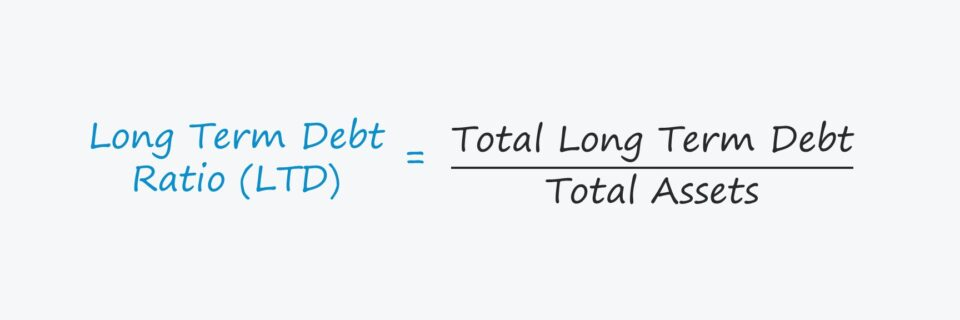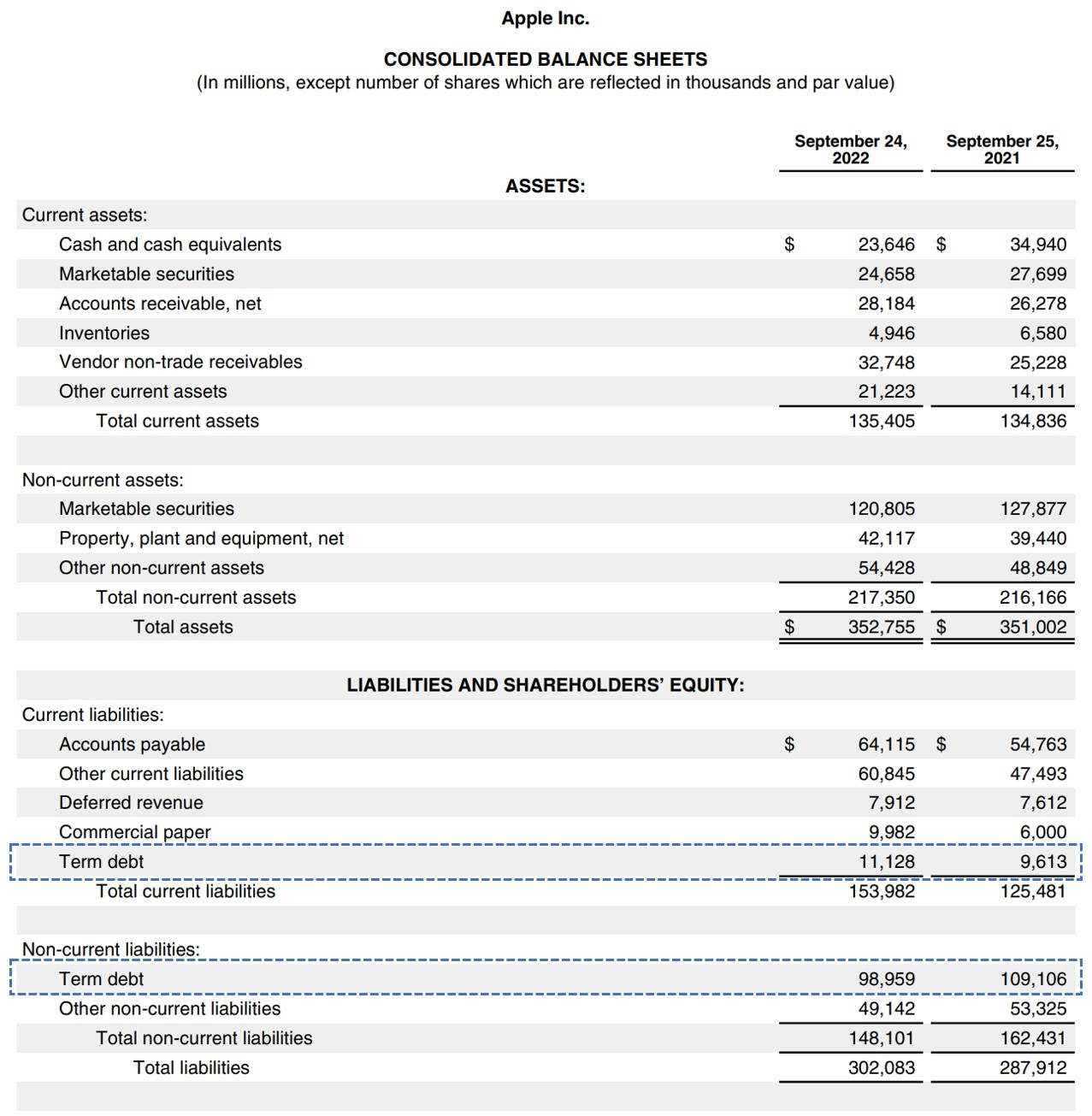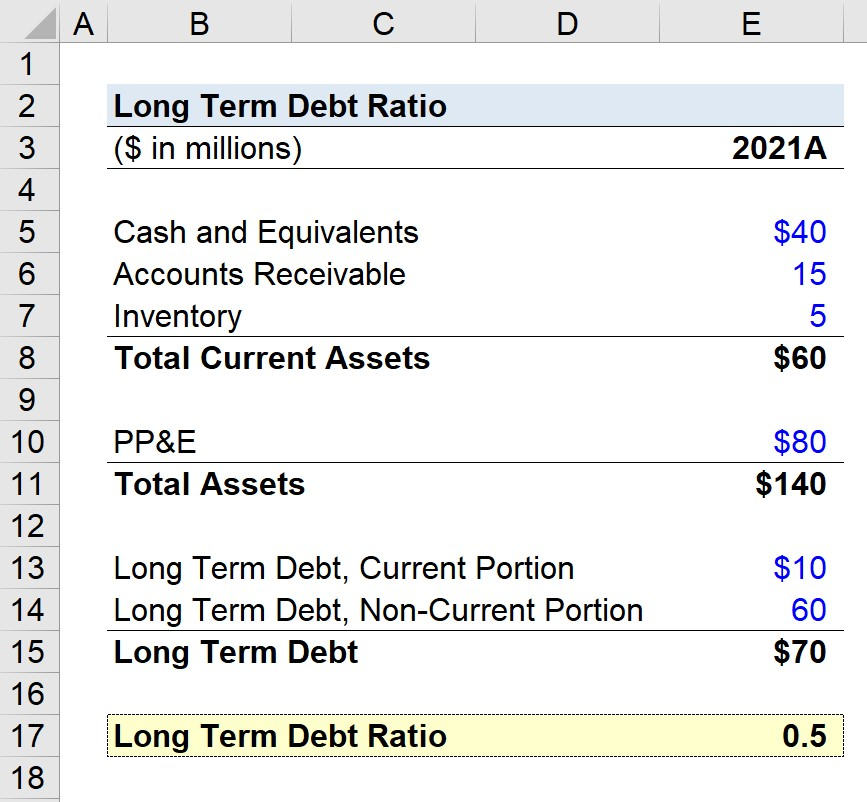Welcome to Wall Street Prep! Use code at checkout for 15% off.# Long Term Debt (LTD)

Guide to Understanding Long Term Debt (LTD)## What is the Definition of Long Term Debt (LTD)?

The “Long Term Debt” line item is recorded in the liabilities section of the balance sheet and represents the borrowings of capital by a company.

Capital is necessary to fund a company’s day-to-day operations such as near-term working capital needs and the purchases of fixed assets (PP&E), i.e. capital expenditures (Capex).

The two methods to raise capital to fund the purchase of resources (i.e. assets) are equity and debt.

1. Equity Financing → The issuance of common shares and preferred stock by a company to outside investors, where capital is exchanged for partial ownership in the company’s equity.
2. Debt Financing → The issuance of debt securities such as term loans and corporate bonds that must be repaid at maturity, along with interest expense over the debt’s tenor, mandatory principal amortization, and repayment of the remaining debt principal on the date of maturity if applicable to the specific lending arrangement.

While assets are ordered based on descending liquidity (i.e. the more quickly an asset can be liquidated into cash proceeds, the higher its placement), liabilities are ordered on the basis of how close their maturity dates are.

The liabilities section of the balance sheet is separated into two parts:

1. Current Liabilities → Maturity < 12 Months
2. Non-Current Liabilities → Maturity > 12 Months

Long term debt (LTD) — as implied by the name — is characterized by a maturity date in excess of twelve months, so these financial obligations are placed in the non-current liabilities section.

## Current Portion of Long Term Debt: Balance Sheet Example

The long term debt (LTD) line item is a consolidation of numerous debt securities with different maturity dates.

Since the repayment of the securities embedded within the LTD line item each have different maturities, the repayments occur periodically rather than as a one-time, “lump sum” payment.

Thus, the “Current Liabilities” section can also include the current portion of long term debt, provided that the debt is coming due within the next twelve months.

As a world example, see below Apple’s 10-K for its fiscal year ending 2022, where the two “Term Debt” components are boxed in blue.Apple Balance Sheet (Source: AAPL Form 10-K)

The general convention for treating short term and long term debt in financial modeling is to consolidate the two line items.

The rationale is that the core drivers are identical, so it would be unreasonable to not combine the two or attempt to project them separately.

Hence, our recommendation is to consolidate the two items, so that the ending LTD balance is determined by a single roll-forward schedule.

## How to Calculate Long Term Debt Ratio?

The long term debt ratio measures the percentage of a company’s assets that were financed by long term financial obligations.

Since the LTD ratio indicates the percentage of a company’s total assets funded by long-term financial borrowings, a lower ratio is generally perceived as better from a solvency standpoint (and vice versa).

The LTD ratio is a solvency ratio, rather than a near-term liquidity ratio. Therefore, short term debt securities like a revolver and commercial paper should intuitively be left off.

However, a clear distinction is necessary here between short-term debt (e.g. commercial paper) and the current portion of long term debt.

Short term debt should be kept off — otherwise it is the capitalization ratio, or “total debt to assets” that is calculated, instead of the long term debt ratio.

The maturity of the debt coming due in the near term does not change the fact that it is in fact long term debt.

## Long Term Debt Ratio Formula

The formula to calculate the long-term debt ratio is as follows.

Long Term Debt Ratio = Long Term Debt ÷ Total Assets

The sum of all financial obligations with maturities exceeding twelve months, including the current portion of LTD, is divided by a company’s total assets.

## Long Term Debt Ratio Calculator

We’ll now move on to a modeling exercise, which you can access by filling out the form below.Submitting...

## Long Term Debt Ratio Calculation Example (LTD)

Suppose we’re tasked with calculating the long term debt ratio of a company with the following balance sheet data.

Balance Sheet
(\$ in millions) 2021A
Cash and Equivalents \$40 million
Accounts Receivable (A/R) \$15 million
Inventory \$5 million
Total Current Assets \$60 million
Property, Plant and Equipment (PP&E) \$80 million
Total Assets \$140 million
LTD, Current Portion \$10 million
LTD, Non-Current Portion \$60 million
Total Long Term Debt \$70 million

By dividing the company’s total long term debt — inclusive of the current and non-current portion — by the company’s total assets, we arrive at a long term debt ratio of 0.5.

• Total Assets = \$60 million + \$80 million = \$140 million
• Total Long-Term Debt = \$10 million + \$60 million = \$70 million
• Long-Term Debt Ratio = \$70 million ÷ \$140 million = 0.50

The 0.5 LTD ratio implies that 50% of the company’s resources were financed by long term debt.

Thus, the company has \$0.50 in long term debt (LTD) for each dollar of assets owned.Step-by-Step Online Course

### Everything You Need To Master Financial Modeling

Enroll in The Premium Package: Learn Financial Statement Modeling, DCF, M&A, LBO and Comps. The same training program used at top investment banks.